# 83 Results

View
Selected filters:
• WI.Math.Practice.MP.4
RemixConditional Remix & Share Permitted
CC BY-NC-SA
Rating

Students will create a 3D model comprised of 3 different shapes. This ...

Students will create a 3D model comprised of 3 different shapes. This will be done with Play-doh or in Tinkercad. They will then find the volume and surface area of the composite 3D shape.

Subject:
Mathematics
Material Type:
Lesson
Author:
Lynn Aprill
Nicholas Schaumberg
09/04/2019
Remix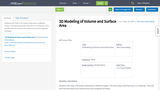Conditional Remix & Share Permitted
CC BY-NC-SA
Rating

Students will create a 3D model comprised of 3 different shapes. This ...

Students will create a 3D model comprised of 3 different shapes. This will be done with Play-doh or in Tinkercad. They will then find the Volume and Surface Area of the composite 3D shape.

Subject:
Education
Material Type:
Lesson
Author:
Nicholas Schaumberg
08/21/2019
Remix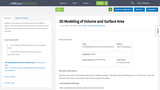Conditional Remix & Share Permitted
CC BY-NC-SA
Rating

Students will create a 3D model comprised of 3 different shapes. This ...

Students will create a 3D model comprised of 3 different shapes. This will be done with Play-doh or in Tinkercad. They will then find the volume and surface area of the composite 3D shape.

Subject:
Mathematics
Material Type:
Lesson
Author:
Lynn Aprill
Nicholas Schaumberg
11/19/2019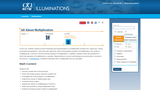Rating

This unit consists of four lessons in which students explore several meanings ...

This unit consists of four lessons in which students explore several meanings and representations of multiplication, including number lines, sets, arrays, and balance beams. They also learn about the commutative property of multiplication, the results of multiplying by 1 and by 0, and the inverse property of multiplication. Students write story problems and create pictographs. The unit includes activity sheets, assessment ideas, links to related applets, reflective questions for students and teachers, extensions and a bibliography of children's literature with a multiplication focus.

Subject:
Mathematics
Algebra
Material Type:
Interactive
Lesson Plan
Provider:
National Council of Teachers of Mathematics
Provider Set:
Illuminations
Author:
Grace M. Burton
11/05/2000Rating

In this data analysis activity students investigate data in connection with recyclable ...

In this data analysis activity students investigate data in connection with recyclable materials and develop plans to help the environment. Students collect data about aluminum can usage and graph that data in a line plot. The lesson includes student worksheet and extension suggestions.

Subject:
Mathematics
Material Type:
Interactive
Lesson Plan
Provider:
National Council of Teachers of Mathematics
Provider Set:
Illuminations
11/05/2008Rating

In this lesson plan students conduct a survey of ten students, collect ...

In this lesson plan students conduct a survey of ten students, collect data in a tally chart, and then display and analyze the results in a bar graph. In the analysis students must compare numbers, add, and subtract using the data in the bar graph to generate questions as well as answer them. The lesson plan includes a graph template, a link to an interactive graphing tool, extension questions, and a fact family sheet to show the related facts from their graph analysis.

Subject:
Mathematics
Material Type:
Interactive
Lesson Plan
Provider:
National Council of Teachers of Mathematics
Provider Set:
Illuminations
Author:
Grace M. Burton
11/05/2008Rating

In this 8-lesson unit students use buttons to explore logical and numerical ...

In this 8-lesson unit students use buttons to explore logical and numerical relationships that form the conceptual basis for understanding addition and subtraction operations. Topics include counting, ordinal numbers (and relative position), classification (attributes), relationships between numbers, addition of sets, commutativity of addition, sums to 10, fact families (including subtraction), three models of subtraction ("take away", comparative, missing addend), and bar graphs. Includes student activity sheets and a link to an online graphing applet.

Subject:
Mathematics
Material Type:
Interactive
Lesson Plan
Provider:
National Council of Teachers of Mathematics
Provider Set:
Illuminations
Author:
Grace M. Burton
11/05/2000Rating

In this Illuminations lesson plan students explore the use of variables as ...

In this Illuminations lesson plan students explore the use of variables as they solve for the weights of objects using information presented in pictures. Learners model situations that involve adding and subtracting whole numbers, using objects, pictures, and symbols. A student activity sheet is available and downloadable (pdf).

Subject:
Mathematics
Algebra
Material Type:
Interactive
Lesson Plan
Provider:
National Council of Teachers of Mathematics
Provider Set:
Illuminations
11/05/2009Rating

This interactive applet introduces students to the topic of combinations, a basic ...

This interactive applet introduces students to the topic of combinations, a basic concept in probability. Users create combinations of shirts and pants to determine the total number of possible outfits. They may simply explore by placing the clothes on Bobbie, or make a guess and then test it. The number of shirt and pants choices is customizable. An optional voice provides prompts and feedback.

Subject:
Mathematics
Material Type:
Interactive
Lesson Plan
Provider:
National Council of Teachers of Mathematics
Provider Set:
Illuminations
11/05/2000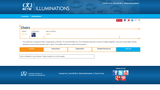Rating

This Java applet activity allows students to explore the various situations described ...

This Java applet activity allows students to explore the various situations described in "The Chairs Around the Table" lesson (cataloged separately). The user can select Exploration mode, in which the number of chairs needed for a particular arrangement of tables is displayed; or Guess, in which the user is able to construct an arrangement and then predict the number of chairs. There are two types of tables to choose from and two different table arrangements. Instructions and exploration question are provide.

Subject:
Mathematics
Algebra
Functions
Material Type:
Interactive
Lesson Plan
Provider:
National Council of Teachers of Mathematics
Provider Set:
Illuminations
11/05/2011Rating

In this lesson from Illuminations, students explore and discover linear relationships. Linear ...

In this lesson from Illuminations, students explore and discover linear relationships. Linear patterns are identified, extended and described verbally, numerically and algebraically through three investigations. Using manipulatives and the linked applet, "Chairs", learners determine the number of chairs needed when the number of tables is known, and vice versa. Instructional plan, questions for the students, assessment options, extensions and teacher reflections are provided.

Subject:
Mathematics
Algebra
Functions
Material Type:
Interactive
Lesson Plan
Provider:
National Council of Teachers of Mathematics
Provider Set:
Illuminations
Author:
Samuel E. Zordak
11/05/2011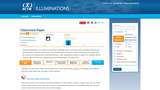Rating

In this data collection and analysis activity students investigate data in connection ...

In this data collection and analysis activity students investigate data in connection with recyclable materials and develop plans to help the environment. This activity requires students to keep track of their own paper use for a week, graph the data with a line graph and then interpret their results with a partner. The lesson includes student worksheets and extension suggestions.

Subject:
Mathematics
Material Type:
Interactive
Lesson Plan
Provider:
National Council of Teachers of Mathematics
Provider Set:
Illuminations
11/05/2008Rating

This lesson develops number skills, understanding of coin values, and the concept ...

This lesson develops number skills, understanding of coin values, and the concept of equivalence. Students use coin blocks, concrete representations of the value of coins (pennies, nickels, dimes, and quarters), to compose and decompose values up to a dollar. The resource includes a 3-minute video for teachers on how to build the coin blocks prior to the lesson. An interactive game, Coin Box, provides additional practice for students and is cataloged separately.

Subject:
Mathematics
Material Type:
Interactive
Lesson Plan
Provider:
National Council of Teachers of Mathematics
Provider Set:
Illuminations
Author:
Jennifer Rising
11/05/2011Rating

This 2-lesson unit focuses on combinations, a subject related to probability. Students ...

This 2-lesson unit focuses on combinations, a subject related to probability. Students develop strategies for discovering all the possible combinations in two given situations. They learn to collect and organize data and then use the results to generalize methods for determining possible combinations. They discuss how the number of possible outcomes is affected by decisions about the order of choices, or whether choices may be repeated. The unit includes student activity sheets, questions and extensions for students, and a link to an interactive applet.

Subject:
Mathematics
Material Type:
Interactive
Lesson Plan
Provider:
National Council of Teachers of Mathematics
Provider Set:
Illuminations
Author:
Marcy Cook (adapted from "Ideas: Combinations", The Arithmetic Teacher Vol. 36, No. 1, September 1988)
11/05/2000Rating

In this lesson plan students learn to create bar graphs using unifix ...

In this lesson plan students learn to create bar graphs using unifix cubes, translate this representation to graph paper, and then compare the data that has been collected and displayed. Students are encouraged to make up their own questions about the data (favorite juice) and to practice with multiple sets of data. Sample questions for students and extension ideas are included. The lesson contains links to a PDF of graph paper and the Bar Grapher tool which is cataloged separately in this database.

Subject:
Mathematics
Material Type:
Interactive
Lesson Plan
Provider:
National Council of Teachers of Mathematics
Provider Set:
Illuminations
Author:
Grace M. Burton
11/05/2012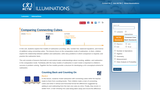Rating

In this 6-lesson unit, students explore 5 models of subtraction (counting, sets, ...

In this 6-lesson unit, students explore 5 models of subtraction (counting, sets, number line, balanced equations, and inverse of addition) using connecting cubes. The lesson activities focus on the comparative mode of subtraction as children investigate the relationship between addition and subtraction, write story problems in which comparison is required, and practice the subtraction facts. The lessons include printable student activity sheets, a bibliography of children's counting books, questions for student discussion and teacher reflection, assessment options, extensions, and links to online applets (cataloged separately).

Subject:
Mathematics
Material Type:
Interactive
Lesson Plan
Provider:
National Council of Teachers of Mathematics
Provider Set:
Illuminations
Author:
Grace M. Burton
11/05/2012Only Sharing Permitted
CC BY-NC-ND
Rating

This lesson unit is intended to help teachers assess how well students ...

This lesson unit is intended to help teachers assess how well students are able to interpret exponential and linear functions and in particular to identify and help students who have the following difficulties: translating between descriptive, algebraic and tabular data, and graphical representation of the functions; recognizing how, and why, a quantity changes per unit intervale; and to achieve these goals students work on simple and compound interest problems.

Subject:
Algebra
Measurement and Data
Material Type:
Assessment
Lesson Plan
Provider:
Shell Center for Mathematical Education
U.C. Berkeley
Provider Set:
Mathematics Assessment Project (MAP)
Mathematics Assessment Project (MAP)
11/01/2017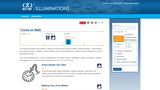Rating

This unit contains two lessons which help students develop number sense through ...

This unit contains two lessons which help students develop number sense through activities involving collection, representation, and analysis of data. Students also practice reading and writing large numbers and develop estimation skills. In Lesson 1, Every Breath You Take, students estimate the number of breaths taken during a specified time, experiment, and display real-life data. In Lesson 2, Making Your First Millions, students develop the concept of a million by working with smaller numerical units, such as blocks of 10 or 100, and then expanding the idea by multiplication or repeated addition. They analyze situations and identify patterns that will enable them to develop the concept of large numbers. Each lesson includes student activity sheets, an instructional plan, and extensions.

Subject:
Mathematics
Material Type:
Interactive
Lesson Plan
Provider:
National Council of Teachers of Mathematics
Provider Set:
Illuminations
Author:
William R. Speer and Daniel J. Brahier
11/05/2000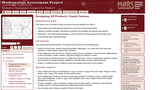Only Sharing Permitted
CC BY-NC-ND
Rating

This lesson unit is intended to help teachers assess how well students ...

This lesson unit is intended to help teachers assess how well students are able to: Select appropriate mathematical methods to use for an unstructured problem; interpret a problem situation, identifying constraints and variables, and specify assumptions; work with 2- and 3-dimensional shapes to solve a problem involving capacity and surface area; and communicate their reasoning clearly.

Subject:
Geometry
Material Type:
Assessment
Lesson Plan
Provider:
Shell Center for Mathematical Education
U.C. Berkeley
Provider Set:
Mathematics Assessment Project (MAP)
Mathematics Assessment Project (MAP)
07/26/2017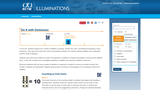Rating

In this 6-lesson unit, students use dominoes to explore four models of ...

In this 6-lesson unit, students use dominoes to explore four models of addition: counting, number line, sets, and balanced equations. They learn about the commutative property, the relation between addition and subtraction, the result of adding 0, and the concept of doubles. Students write story problems which involve the operation of addition and begin to memorize the addition facts. They represent addition in pictures. The various models of addition help students develop a rich conceptual schema for addition. Included are a Bibliography of Counting Books, student materials, questions for student and teacher reflection, assessment and extension ideas. [Suggestion: Use the alternate applet, Pan Balance - Numbers, listed as a Related Resource, rather than Pan Balance - Shapes, in Lesson 4.]

Subject:
Mathematics
Material Type:
Interactive
Lesson Plan
Provider:
National Council of Teachers of Mathematics
Provider Set:
Illuminations
Author:
Grace M. Burton